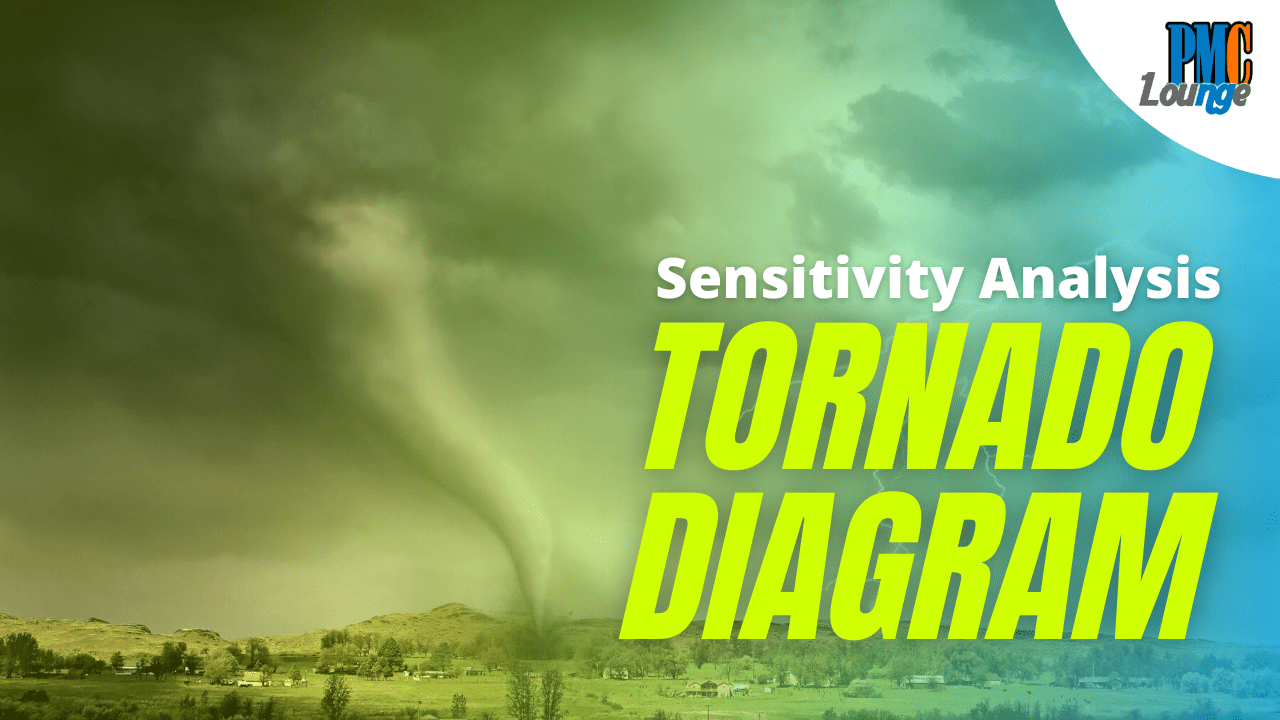# Sensitivity Analysis using Tornado DiagramsWe touched a bit on the topic of sensitivity analysis when we started off with the Perform Quantitative Risk Analysis process.

Sensitivity Analysis is where you look at one variable or risk factor in complete isolation and analyze the impact it might have on the project.

Let’s say you want to do a sensitivity analysis of the risk of procurement delay. You will only consider the delay it will have on your project while assuming the rest of the project is going as per the plan.

Tornado diagrams are used to represent a project’s sensitivity to each risk factor in isolation. You can achieve this by concentrating on one uncertain factor while assuming that the rest of the project moves as per the plan.

For example, the table below lists all the risks for a project along with their quantitative analysis,

Now while we have the P x I value of each risk, it is not necessary that risks follow the absolute values. Risks can have a range. For instance, ‘Procurement delays’ can cause a schedule delay of 2 weeks or 2 months and that is why a 0.7 impact might not represent the true picture.

This is where Tornado Diagram comes in handy. It represents the ‘Procurement delays’ as well as other risks in a range.

In the diagram above, we have reserved \$60,000 for risks and the procurement delays, can cost anywhere from \$10K to \$90K. This range of \$10K to \$90K is the sensitivity of the risk.

The name ‘Tornado’ diagram comes from the fact that the diagram does look like a tornado.

Check more articles on Risk Management